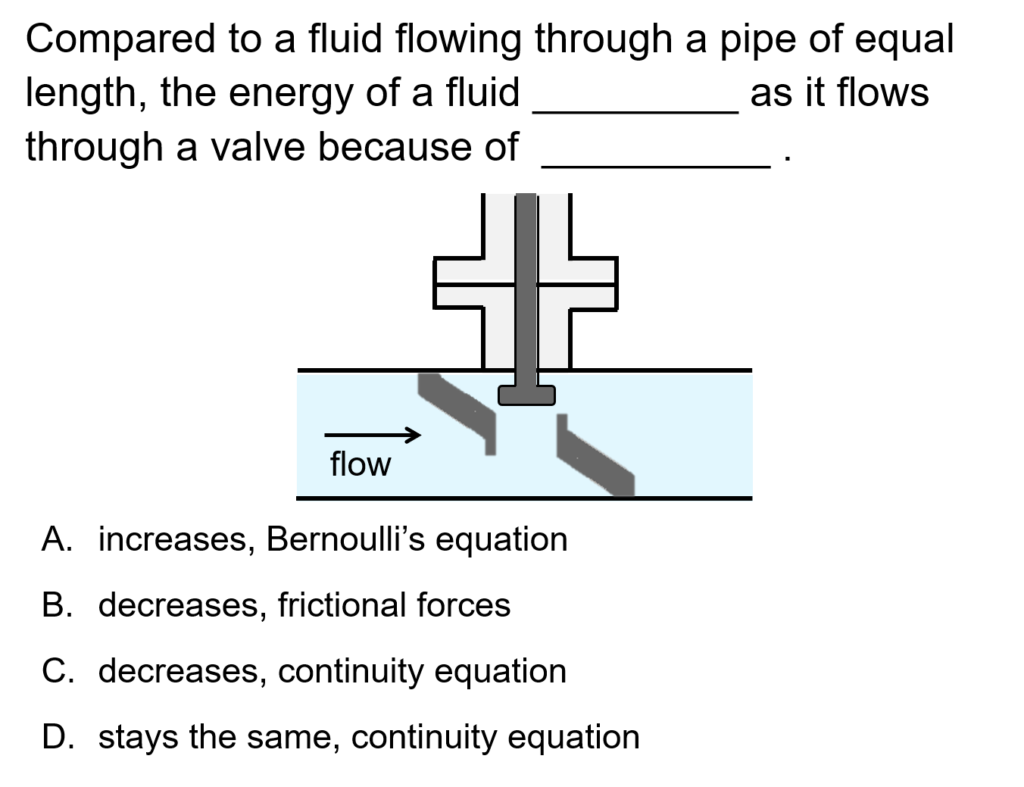#### Calculating Length and Equivalent Length in a Pipe: ConcepTest and Example Problem

Try to answer this ConcepTest and solve the example problem before using this module. Studies show that trying to answer the questions before studying material improves learning and retention. We suggest that you write down the reasons for your answers. By the end of this module, you should be able to answer these on your own. Answers will be given at the end of this module.##### Example Problem 1

Consider a galvanized iron pipe of 0.075 m diameter with a 90° threaded elbow. What is the equivalent length? How would this change if the elbow is flanged? Assume the flow is wholly turbulent.